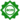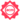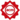Grammar-based test generators are highly efficient in producing syntactically valid test inputs, and give their user precise control over which test inputs should be generated. Adapting a grammar or a test generator towards a particular testing goal can be tedious, though. We introduce the concept of a grammar transformer, specializing a grammar towards inclusion or exclusion of specific patterns: “The phone number must not start with 011 or +1”. To the best of our knowledge, ours is the first approach to allow for arbitrary Boolean combinations of patterns, giving testers unprecedented flexibility in creating targeted software tests. The resulting specialized grammars can be used with any grammar-based fuzzer for targeted test generation, but also as validators to check whether the given specialization is met or not, opening up additional usage scenarios. In our evaluation on real-world bugs, we show that specialized grammars are accurate both in producing and validating targeted inputs.

In the paper, for negation of a rule such as below

<A X> ::= <B E> <C F> <D>


we provide the following result

<A neg(X)> ::= <B neg(E)> <C F> <D>
| <B E> <C neg(F)> <D>


However, this expansion is wrong. The reason is that our guarantee is simply that X exists in one of <B E> or <C F> or <D>. The above result assumes that all the specializations in tokens are necessary to reproduce the fault. However, this is incorrect. In particular, the fault may be present either in <B E> or <C F> or even in <D>.

There are two choices here. The first (and recommended option) is to simplify the expression first so that negation is pushed to the innermost elements of the expression X. That is, if we are trying to evaluate say <A neg(and(A,B))> then, simplify it to <A or(neg(A),neg(B))> first. Next, produce the nonterminal corresponding to <A neg(B)> as we describe here. Use that for constructing <A or(neg(A),neg(B))>. The idea described there in summary is to (1) construct the nonreaching grammar (similar to reaching grammar in the paper, but opposite), then negate the pattern grammar, and merge both grammars. This is recommended both because it is simpler, and is computationally less demanding.

The second choice is to construct the negation directly for the expression. The idea is similar to the first choice. Here, we start by noticing that we need an equivalent of nonreaching grammar for the entire expression. Hence, we take the base grammar, and produce an nonreaching nonterminal thus.

Given

<A> ::= <B> <C> <D>


The nonreaching nonterminal <A neg(E&F)> would have

<A neg(E&F)> ::= <B neg(E&F)> <C neg(E&F)> <D neg(E&F)>


and so on for each rule of <A>. The complication is when such an expression can be constructed by components. For example, if there is a rule in <A E&F> as below. To resolve this issue, we use the basic rule. To negate a rule, the idea is to generate all rules that will not match the derivation trees generated by that rule. With this in mind, negating a rule that contains components of the original expression means to generate all rules such that each generated rule just misses being able to generate a matching tree. So, given

<A E&F> ::= <B E> <C F> <D>


Then, negation would be

<A neg(E&F)> ::= <B neg(E)> <C F> <D neg(E&F)>
| <B E> <C neg(F)> <D neg(E&F)>


Each just missing being able to generate a fault of kind E&F.

If <D> can’t embed E or F, then this will simplify to

<A neg(E&F)> ::= <B neg(E)> <C F> <D>
| <B E> <C neg(F)> <D>


What happens for pattern rules which require an exact match? We note that pattern grammars are essentially conjunction (&) patterns with extra height constraints, and are handled like above.

Artifacts available(implies functionaland reusableat ICSE)